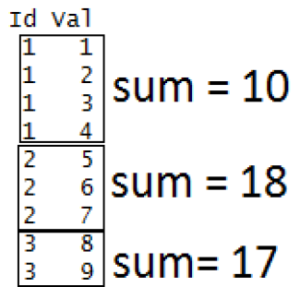# Looping over Objects in R Programming

Prerequisite: Data Structures in R Programming

One of the biggest issues with the “for” loop is its memory consumption and its slowness in executing a repetitive task. And when it comes to dealing with large data set and iterating over it, for loop is not advised. R provides many alternatives to be applied to vectors for looping operations that are pretty useful when working interactively on a command line. In this article, we deal with `apply()` function and its variants:

``` apply() lapply() tapply() mapply() ```

Let us see what each of these functions does.

Looping Function Operation
`apply()` Applies a function over the margins of an array or matrix
`lapply()` Apply a function over a list or a vector
`tapply()` Apply a function over a ragged array
`mapply()` Multivariate version of `lapply()`
• `apply()`: This function applies a given function over the margins of a given array.

apply(array, margins, function, …)

array = list of elements
margins = dimension of the array along which the function needs to be applied
function = the operation which you want to perform

Example:

 `# R program to illustrate ` `# apply() function ` ` `  `# Creating a matrix ` `A ``=` `matrix(``1``:``9``, ``3``, ``3``) ` `print``(A) ` ` `  `# Applying apply() over row of matrix ` `# Here margin 1 is for row  ` `r ``=` `apply``(A, ``1``, ``sum``) ` `print``(r) ` ` `  `# Applying apply() over column of matrix ` `# Here margin 2 is for column ` `c ``=` `apply``(A, ``2``, ``sum``) ` `print``(c) `

Output:

```[, 1] [, 2] [, 3]
[1, ]    1    4    7
[2, ]    2    5    8
[3, ]    3    6    9

 12 15 18
  6 15 24
```
• `lapply():` This function is used to apply a function over a list. It always returns a list of the same length as the input list.

lapply(list, function, …)

list = Created list
function = the operation which you want to perform

Example:

 `# R program to illustrate ` `# lapply() function ` ` `  `# Creating a matrix ` `A ``=` `matrix(``1``:``9``, ``3``, ``3``) ` ` `  `# Creating another matrix ` `B ``=` `matrix(``10``:``18``, ``3``, ``3``)  ` ` `  `# Creating a list ` `myList ``=` `list``(A, B) ` ` `  `# applying lapply() ` `determinant ``=` `lapply(myList, det) ` `print``(determinant) `

Output:

```[]
 0

[]
 5.329071e-15
```
• `tapply()`: This function is used to apply a function over subset of vectors given by a combination of factors.

tapply(vector, factor, function, …)

vector = Created vector
factor = Created factor
function = the operation which you want to perform

Example:

 `# R program to illustrate ` `# tapply() function ` ` `  `# Creating a factor ` `Id` `=` `c(``1``, ``1``, ``1``, ``1``, ``2``, ``2``, ``2``, ``3``, ``3``) ` ` `  `# Creating a vector ` `val ``=` `c(``1``, ``2``, ``3``, ``4``, ``5``, ``6``, ``7``, ``8``, ``9``) ` ` `  `# applying tapply() ` `result ``=` `tapply(val, ``Id``, ``sum``) ` `print``(result) `

Output:

```1  2  3
10 18 17
```

How does the above code work?• `mapply()`: It’s a multivariate version of `lapply()`. This function can be applied over several list simultaneously.

mapply(function, list1, list2, …)

function = the operation which you want to perform

list1, list2 = Created lists

Example:

 `# R program to illustrate ` `# mapply() function ` ` `  `# Creating a list ` `A ``=` `list``(c(``1``, ``2``, ``3``, ``4``)) ` ` `  `# Creating another list ` `B ``=` `list``(c(``2``, ``5``, ``1``, ``6``)) ` ` `  `# Applying mapply() ` `result ``=` `mapply(``sum``, A, B) ` `print``(result) `

Output:

``` 24
```

My Personal Notes arrow_drop_upCheck out this Author's contributed articles.

If you like GeeksforGeeks and would like to contribute, you can also write an article using contribute.geeksforgeeks.org or mail your article to contribute@geeksforgeeks.org. See your article appearing on the GeeksforGeeks main page and help other Geeks.

Please Improve this article if you find anything incorrect by clicking on the "Improve Article" button below.

Article Tags :

1

Please write to us at contribute@geeksforgeeks.org to report any issue with the above content.